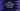# Python program to count the frequency of each word in a string## Python program to count the frequency of each word in a string :

In this python tutorial, we will learn how to count the frequency of each word in a user input string. The program will read all words, find out the number of occurrences for each word and print them out. It will also sort all the words alphabetically.

To solve this problem, we will use one dictionary. Dictionary is an unordered and mutable collection. It stores data as key-value pairs. Using any key, we can access its value. We can even modify the value for a specific key.

A python dictionary is written using a curly bracket. Each key and value are separated using colon (:), and all key-value pairs are separated with a comma (,).

We will use one dictionary to store the frequency of the word in a string. For this dictionary, the keys will be the words of the string, and the values will be the frequency for that word. For the string “hello world hello”, it will look as like below :

``````key - hello , value - 2
key - world , value - 1``````

As you can see, the word ‘hello’ appeared two times in the string. So, the value is 2 for the key ‘hello’. Similarly, for the key ‘world’, value is 1.

Also, it will print the value of the world before hello i.e. alphabetically.

### Algorithm :

The algorithm for the above problem is like below :

1. Ask the user to enter the string. Store it in a variable.
2. Create one dictionary to store the frequency of each word in the string.
3. Read the words in the string one by one.
4. For each word, check if the dictionary has any key equal to the current word. If yes, increment the value for that key by 1. If not, add one new key-value pair with key equal to the word and value as 1.
5. Sort all keys in the dictionary alphabetically.
6. Finally, print out the frequency of each word to the user.

Let’s take a look at the program :

### Python program :

``````#1
input_line = input("Enter a string : ")

#2
words_dict = {}

#3
for word in input_line.split():
words_dict[word] = words_dict.get(word,0) + 1

#4
for key in sorted(words_dict):
print("{} : {}".format(key,words_dict[key]))``````

The source code is shared on Github here.

### Explanation :

The commented numbers in the above program denote the step-number below :

1. Ask the user to enter a string. Read and store it in the input_line variable.
2. Create one dictionary to store the key-value pair, where the key is the word and value is the frequency of that word. This is an empty dictionary. For creating an empty dictionary, we can use one empty curly braces.
3. Start scanning the words of the string one by one. Read the current frequency value for that word from the dictionary and add 1 to it or increment it by 1. If the current frequency is not available, return 0.

Here, we are splitting the string using ‘split()’ method. Python string split() method returns one list holding all the words in the string. Using the for loop, we are iterating through the list items, i.e. iterating the words of the string.

1. Sort all keys of the dictionary alphabetically. That means, sort all words contains in the dictionary alphabetically. The sorted() method is used to sort the keys in the dictionary.

Finally, print out the value of the frequency of each word.